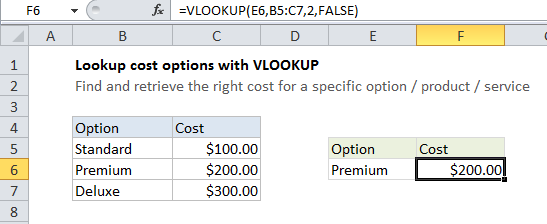## Excel Office

Excel How Tos, Tutorials, Tips & Tricks, Shortcuts

# Lookup up cost for product or service in Excel

This tutorial shows how to Lookup up cost for product or service in Excel using the example below;

## Formula

`=VLOOKUP(product,table,column,FALSE)`## Explanation

If you have a list of products or services (or related options), with associated costs, you can use VLOOKUP to find and retrieve the cost for a specific option.

Worked Example:   VLOOKUP function: Description, Usage, Syntax, Examples and Explanation

In the example shown, the formula in cell F6 is:

`=VLOOKUP(E6,B5:C7,2,FALSE)`

This formula uses the value of cell E6 to lookup and retrieve the right cost in the range B5:C7.

Worked Example:   Self-contained VLOOKUP in Excel

### How this formula works

This is a basic example of VLOOKUP in “exact match” mode. The lookup value is B6, the table is the range B5:C7, the column is 2, and the last argument, FALSE, forces VLOOKUP to do an exact match.

Worked Example:   Merge tables with VLOOKUP in Excel

If VLOOKUP finds a matching value, the associated cost will be retrieved from the 2nd column (column C). If no match is found, VLOOKUP will return the #N/A error.